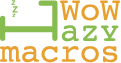# Is my ahk correct for mods?

hello can anyone tell me if my ahk is correct for using mods
1st time it try to make one like this

{
\$1:: ; If 1 is pressed
\$^1:: ; If 1+control is pressed
\$+1:: ; If 1+shift is pressed
\$!1:: ; If 1+alt is pressed
Loop ; If any of the above is true then loop below
{
if not GetKeyState(“1”, “P”) ;
break
if GetKeyState(“LCtrl”, “P”) ;
Send ^e
else if GetKeyState(“LShift”, “P”) ;
Send +e
else if GetKeyState(“LAlt”, “P”) ;
Send !1
else
Send 1 ;
sleep 100 ;
}
return
\$2:: ; If 2 is pressed
\$^2:: ; If 2+control is pressed
\$+2:: ; If 2+shift is pressed
\$!2:: ; If 2+alt is pressed
Loop ; If any of the above is true then loop below
{
if not GetKeyState(“2”, “P”) ;
break
if GetKeyState(“LCtrl”, “P”) ;
Send ^e
else if GetKeyState(“LShift”, “P”) ;
Send +e
else if GetKeyState(“LAlt”, “P”) ;
Send !2
else
Send 2 ;
sleep 100 ;
}
return
}